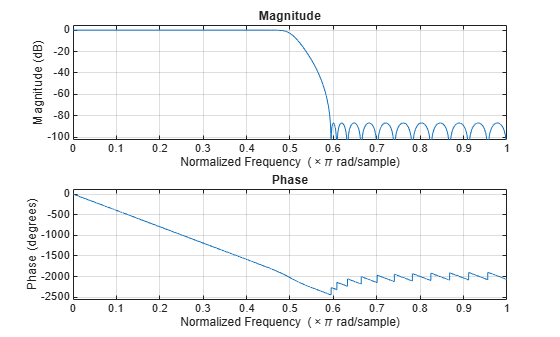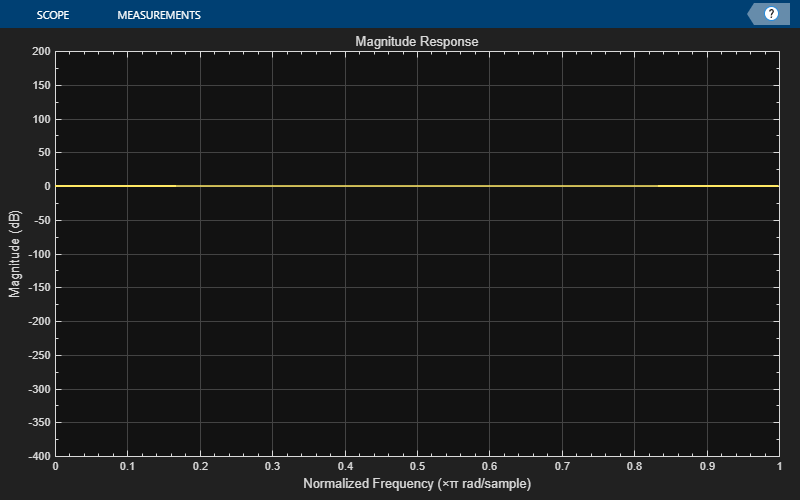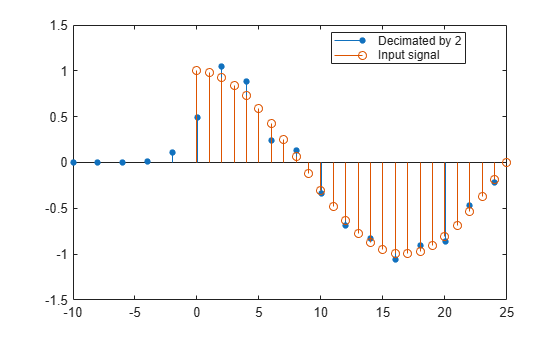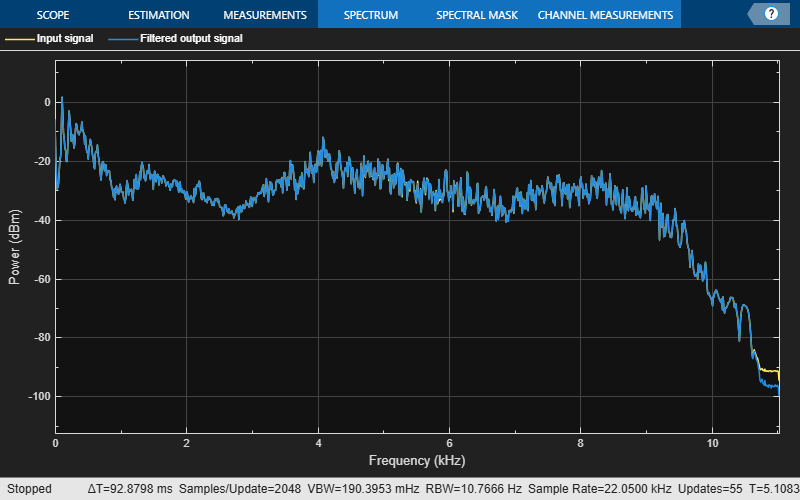# dsp.IIRHalfbandDecimator

Decimate by factor of two using polyphase IIR

## Description

The `dsp.IIRHalfbandDecimator` System object™ performs efficient polyphase decimation of the input signal by a factor of two. To design the halfband filter, you can specify the object to use an elliptic design or a quasi-linear phase design. The object uses these design methods to compute the filter coefficients. To filter the inputs, the object uses a polyphase structure. The allpass filters in the polyphase structure are in a minimum multiplier form.

Elliptic design introduces nonlinear phase and creates the filter using fewer coefficients than quasi linear design. Quasi-linear phase design overcomes phase nonlinearity at the cost of additional coefficients.

Alternatively, instead of designing the halfband filter using a design method, you can specify the filter coefficients directly. When you choose this option, the allpass filters in the two branches of the polyphase implementation can be in a minimum multiplier form or in a wave digital form.

You can also use the `dsp.IIRHalfbandDecimator` object to implement the analysis portion of a two-band filter bank to filter a signal into lowpass and highpass subbands.

To filter and downsample your data:

1. Create the `dsp.IIRHalfbandDecimator` object and set its properties.

2. Call the object with arguments, as if it were a function.

## Creation

### Syntax

``iirhalfbanddecim = dsp.IIRHalfbandDecimator``
``iirhalfbanddecim = dsp.IIRHalfbandDecimator(Name=Value)``

### Description

````iirhalfbanddecim = dsp.IIRHalfbandDecimator` returns a halfband decimator, `iirhalfbanddecim`, with the default settings. Under the default settings, the System object filters and downsamples the input data with a halfband frequency of `22050` Hz, a transition width of `4100` Hz, and a stopband attenuation of `80` dB.```

example

````iirhalfbanddecim = dsp.IIRHalfbandDecimator(Name=Value)` returns an IIR halfband decimator, with additional properties specified by one or more `Name-Value` pair arguments.Example: ```iirhalfbanddecim = dsp.IIRHalfbandDecimator(Specification="Filter order and stopband attenuation")``` creates an IIR halfband decimator object with filter order set to `9` and stopband attenuation set to `80` dB.```

## Properties

expand all

Unless otherwise indicated, properties are nontunable, which means you cannot change their values after calling the object. Objects lock when you call them, and the `release` function unlocks them.

If a property is tunable, you can change its value at any time.

### Main Properties

Filter design parameters, specified as a character vector. When you set `Specification` to one of the filter design options, you can specify the filter design parameters using the corresponding `FilterOrder`, `StopbandAttenuation`, and `TransitionWidth` properties. Also, you can specify the design method using `DesignMethod`. When you set `Specification` to `"Coefficients"`, you can specify the coefficients directly.

Order of the IIR halfband filter, specified as a positive scalar integer. If you set `DesignMethod` to `"Elliptic"`, then `FilterOrder` must be an odd integer greater than one. If you set `DesignMethod` to `"Quasi-linear phase"`, then `FilterOrder` must be a multiple of four.

#### Dependencies

To enable this property, set `Specification` to `"Filter order and stopband attenuation"` or ```"Filter order and transition width"```.

Data Types: `single` | `double` | `int8` | `int16` | `int32` | `int64` | `uint8` | `uint16` | `uint32` | `uint64`

Minimum attenuation needed in the stopband of the IIR halfband filter, specified as a positive real scalar. Units are in dB.

#### Dependencies

To enable this property, set `Specification` to `"Filter order and stopband attenuation"` or ```"Transition width and stopband attenuation"```.

Data Types: `single` | `double`

Transition width of the IIR halfband filter, specified as a positive real scalar or in normalized frequency units (since R2023b).

If you set the `NormalizedFrequency` property to:

• `false` –– The value of the transition width is in Hz and must be less than half the `SampleRate` property value.

• `true` –– The value of the transition width is in normalized frequency units. The value must be a positive scalar less than `1.0`.

When you set the `NormalizedFrequency` property to `true` while creating the object and you do not set the transition width, the object sets the default transition width to normalized frequency units using the default sample rate of 44100 Hz.

When you set the `NormalizedFrequency` property to `true` after you create the object, you must specify the transition width in normalized units before you run the object algorithm. To specify the normalized frequency value, set `NormalizedFrequency` to `true` and manually convert the frequency value in Hz to the normalized value using the input sample rate in Hz. For example, if the input sample rate Fs is 44100 Hz, the corresponding transition width value in normalized units is TWHz/(Fs/2).

```iirhalfbanddecim = dsp.IIRHalfbandDecimator; iirhalfbanddecim.NormalizedFrequency = true; iirhalfbanddecim.TransitionWidth = 4100/(44100/2)```

(since R2023b)

#### Dependencies

To enable this property, set `Specification` to `"Transition width and stopband attenuation"` or ```"Filter order and transition width"```.

Data Types: `single` | `double`

Design method for the IIR halfband filter, specified as `"Elliptic"` or `"Quasi-linear phase"`. When you set this property to `"Quasi-linear phase"`, the first branch of the polyphase structure is a pure delay, which results in an approximately linear phase response.

#### Dependencies

To enable this property, set `Specification` to any accepted value except `"Coefficients"`.

Since R2023b

Flag to set frequencies in normalized units, specified as one of these values:

• `true` –– The transition width must be in the normalized frequency units and less than `1.0`.

When you set the `NormalizedFrequency` property to `true` while creating the object and you do not set the transition width, the object sets the default transition width to normalized frequency units using the default sample rate of 44100 Hz.

When you set the `NormalizedFrequency` property to `true` after you create the object, you must specify the transition width in normalized units before you run the object algorithm. To specify the normalized frequency value, set `NormalizedFrequency` to `true` and manually convert the frequency value in Hz to the normalized value using the input sample rate in Hz. For example, if the input sample rate Fs is 44100 Hz, the corresponding transition width value in normalized units is TWHz/(Fs/2).

```iirhalfbanddecim = dsp.IIRHalfbandDecimator; iirhalfbanddecim.NormalizedFrequency = true; iirhalfbanddecim.TransitionWidth = 4100/(44100/2)```

• `false` –– The transition width is in Hz. You can specify the input sample rate through the `SampleRate` property.

#### Dependency

To enable this property, set `Specification` to any accepted value except `"Coefficients"`.

Data Types: `logical`

Input sample rate in Hz, specified as a positive real scalar.

#### Dependency

To enable this property, set:

• `Specification` to any accepted value except `"Coefficients"`.

• `NormalizedFrequency` to `false`. (since R2023b)

Data Types: `single` | `double`

Internal allpass filter implementation structure, specified as ```"Minimum multiplier"``` or `"Wave Digital Filter"`.

This property is not tunable.

#### Dependencies

To enable this property, set `Specification` to `"Coefficients"`. Each structure uses a different coefficients set, independently stored in the corresponding object property.

Allpass polynomial filter coefficients of the first branch, specified as an N-by-`1` or N-by-`2` matrix. N is the number of first-order or second-order allpass sections.

Tunable: Yes

#### Dependencies

To enable this property, set `Specification` to `"Coefficients"` and `Structure` to `"Minimum multiplier"`.

Data Types: `single` | `double` | `int8` | `int16` | `int32` | `int64` | `uint8` | `uint16` | `uint32` | `uint64`

Allpass polynomial filter coefficients of the second branch, specified as an N-by-`1` or N-by-`2` matrix. N is the number of first-order or second-order allpass sections.

Tunable: Yes

#### Dependencies

To enable this property, set `Specification` to `"Coefficients"` and `Structure` to `"Minimum multiplier"`.

Data Types: `single` | `double` | `int8` | `int16` | `int32` | `int64` | `uint8` | `uint16` | `uint32` | `uint64`

Allpass filter coefficients of the first branch in Wave Digital Filter form, specified as an N-by-`1` or N-by-`2` matrix. N is the number of first-order or second-order allpass sections. Each element must have an absolute value less than or equal to `1`.

This property is not tunable.

#### Dependencies

To enable this property, set `Specification` to `"Coefficients"` and `Structure` to `"Wave Digital Filter"`.

Data Types: `single` | `double` | `int8` | `int16` | `int32` | `int64` | `uint8` | `uint16` | `uint32` | `uint64`

Allpass filter coefficients of the second branch in Wave Digital Filter form, specified as the comma-separated pair consisting of `'WDFCoefficients2'` and a N-by-`1` or N-by-`2` matrix. N is the number of first-order or second-order allpass sections. Each element must have an absolute value less than or equal to 1.

This property is not tunable.

#### Dependencies

To enable this property, set `Specification` to `"Coefficients"` and `Structure` to `"Wave Digital Filter"`.

Data Types: `single` | `double` | `int8` | `int16` | `int32` | `int64` | `uint8` | `uint16` | `uint32` | `uint64`

Flag to make the first allpass branch a delay, specified as a logical scalar. When this property is true, the first branch is treated as a pure delay and the properties `AllpassCoefficients1` and `WDFCoefficients1` do not apply.

This property is not tunable.

#### Dependencies

To enable this property, set `Specification` to `"Coefficients"`.

Length of the first branch delay, specified as a finite positive scalar. The value of this property specifies the number of samples by which you can delay the input to the first branch.

This property is not tunable.

#### Dependencies

To enable this property, set `Specification` to `"Coefficients"` and `HasPureDelayBranch` to 1.

Data Types: `single` | `double`

Option to treat the last section of the second branch as first order, specified as a logical scalar. When this property is 1 and the coefficients of the second branch are in an N-by-2 matrix, the object ignores the second element of the last row of the matrix. The last section of the second branch then becomes a first-order section. When this property is set to `0`, the last section of the second branch is a second-order section. When the coefficients of the second branch are in an N-by-1 matrix, this property is ignored.

This property is not tunable.

#### Dependencies

To enable this property, set `Specification` to `"Coefficients"`.

### Code Generation Properties

Allow arbitrary frame length for fixed-size input signals in the generated code, specified as `true` or `false`. When you specify:

• `true` –– The input frame length does not have to be a multiple of the decimation factor 2. The output of the object in the generated code is a variable-size array.

• `false` –– The input frame length must be a multiple of the decimation factor 2.

When you specify variable-size signals, the input frame length can be arbitrary and the object ignores this property in the generated code. When you run this object in MATLAB®, the object supports arbitrary input frame lengths for fixed-size and variable-size signals and this property does not affect the object behavior.

Data Types: `logical`

## Usage

### Syntax

``ylow = iirhalfbanddecim(x)``
``[ylow,yhigh] = iirhalfbanddecim(x)``

### Description

example

````ylow = iirhalfbanddecim(x)` filters the input signal, `x`, using the IIR halfband filter, `iirhalfbanddecim`, and downsamples the output by a factor of 2.```

example

````[ylow,yhigh] = iirhalfbanddecim(x)` computes the `ylow` and `yhigh`, of the analysis filter bank, `iirhalfbanddecim` for input `x`. A Ki-by-N input matrix is treated as N independent channels. The System object generates two power-complementary output signals by adding and subtracting the two polyphase branch outputs respectively. `ylow` and `yhigh` are of the same size and data type.```

### Input Arguments

expand all

Data input, specified as a column vector or a matrix. If the input is a matrix, each column is treated as an independent channel.

The number of rows in the input signal Ki can be arbitrary and does not have to be a multiple of 2. (since R2023b)

This object supports variable-size input signal, that is, the frame length (number of rows) of the signal can change even when the object is locked. However, the number of channels (columns) must remain constant.

Data Types: `single` | `double`
Complex Number Support: Yes

### Output Arguments

expand all

Lowpass subband of decimator output, returned as a column vector or a matrix. The output, `ylow` is a lowpass halfband filtered and downsampled version of the input `x`. Due to the halfband nature of the filter, the downsampling factor is always 2.

When the input is of size Ki-by-N, and Ki is not a multiple of 2, the lowpass subband has an upper bound size of `ceil`(Ki/2)-by-N. (since R2023b)

If Ki is a multiple of 2, then the lowpass subband is of size (Ki/2)-by-N. The number of channels (columns) does not change.

Data Types: `single` | `double`
Complex Number Support: Yes

Highpass subband of decimator output, returned as a column vector or a matrix. The output, `yhigh` is a highpass halfband filtered and downsampled version of the input `x`. Due to the halfband nature of the filter, the downsampling factor is always 2.

When the input is of size Ki-by-N, and Ki is not a multiple of 2, the highpass subband has an upper bound size of `ceil`(Ki/2)-by-N. (since R2023b)

If Ki is a multiple of 2, then the highpass subband is of size (Ki/2)-by-N. The number of channels (columns) does not change.

Data Types: `single` | `double`
Complex Number Support: Yes

## Object Functions

To use an object function, specify the System object as the first input argument. For example, to release system resources of a System object named `obj`, use this syntax:

`release(obj)`

expand all

 `freqz` Frequency response of discrete-time filter System object `fvtool` Visualize frequency response of DSP filters `info` Information about filter System object `cost` Estimate cost of implementing filter System object `polyphase` Polyphase decomposition of multirate filter `outputDelay` Determine output delay of single-rate or multirate filter
 `step` Run System object algorithm `release` Release resources and allow changes to System object property values and input characteristics `reset` Reset internal states of System object

## Examples

collapse all

Create a minimum-order lowpass IIR halfband decimation filter. The filter has a transition width of 0.1859 in normalized frequency units and a stopband attenuation of 80 dB.

```IIRHalfbandDecim = dsp.IIRHalfbandDecimator(... NormalizedFrequency=true,... TransitionWidth=0.1859,... DesignMethod='Quasi-linear phase')```
```IIRHalfbandDecim = dsp.IIRHalfbandDecimator with properties: Main Specification: 'Transition width and stopband attenuation' TransitionWidth: 0.1859 StopbandAttenuation: 80 DesignMethod: 'Quasi-linear phase' NormalizedFrequency: true Use get to show all properties ```

Obtain the filter coefficients.

`c = coeffs(IIRHalfbandDecim);`

Plot the magnitude and phase response.

`fvtool(IIRHalfbandDecim,Analysis='freq')`Use a halfband analysis filter bank and interpolation filter to extract the low frequency subband from a speech signal.

Note: The `audioDeviceWriter` System object™ is not supported in MATLAB Online.

Set up the audio file reader, the analysis filter bank, the audio device writer, and the interpolation filter. The sampling rate of the audio data is 22050 Hz. The halfband filter has an order of 21 and a transition width of 2 kHz.

```afr = dsp.AudioFileReader('speech_dft.mp3',SamplesPerFrame=1024); filterspec = "Filter order and transition width"; Order = 21; TW = 2000; IIRHalfbandDecim = dsp.IIRHalfbandDecimator(... Specification=filterspec,FilterOrder=Order,... TransitionWidth=TW,SampleRate=afr.SampleRate); IIRHalfbandInterp = dsp.IIRHalfbandInterpolator(... Specification=filterspec,FilterOrder=Order,... TransitionWidth=TW,SampleRate=afr.SampleRate/2); ap = audioDeviceWriter(SampleRate=afr.SampleRate);```

View the magnitude response of the halfband filter.

`fvtool(IIRHalfbandDecim)`Read the speech signal from the audio file in frames of 1024 samples. Filter the speech signal into lowpass and highpass subbands with a halfband frequency of 5512.5 Hz. Reconstruct a lowpass approximation of the speech signal by interpolating the lowpass subband. Play the filtered output.

```while ~isDone(afr) audioframe = afr(); xlo = IIRHalfbandDecim(audioframe); ylow = IIRHalfbandInterp(xlo); ap(ylow); end```

Wait until the audio file ends, and then close the input file and release the audio output resource.

```release(afr); release(ap);```

Design an elliptic IIR halfband decimator object of order 31 and a transition width of 0.1 using the `designHalfbandIIR` function. Set the `Verbose` argument to `true`.

```hbIIR = designHalfbandIIR(FilterOrder=31,SystemObject=true,... Structure='decim',Verbose=true)```
```designHalfbandIIR(FilterOrder=31, DesignMethod="butter", Structure="decim", SystemObject=true, Passband="lowpass") ```
```hbIIR = dsp.IIRHalfbandDecimator with properties: Main Specification: 'Coefficients' Structure: 'Minimum multiplier' HasPureDelayBranch: false AllpassCoefficients1: [8x1 double] AllpassCoefficients2: [7x1 double] HasTrailingFirstOrderSection: false Use get to show all properties ```

Create a `dsp.DynamicFilterVisualizer` object and visualize the magnitude response of the filter.

```dfv = dsp.DynamicFilterVisualizer(NormalizedFrequency=true,YLimits=[-400 200]); dfv(hbIIR);```The input is a cosine wave.

```Fs = 1; Fc = 0.03; input = cos(2*pi*Fc*(0:39)'/Fs);```

Decimate the cosine signal using the IIR halfband decimator.

`output = hbIIR(input);`

Plot the original and decimated signals. In order to plot the two signals in the same plot, you must account for the output delay introduced by the IIR halfband decimator and the scaling introduced by the filter. Use the `outputDelay` function to compute the `delay` introduced by the decimator. Shift the output by this delay value.

Visualize the input and the resampled signals. Due to the decimation factor of 2, the output samples coincide with every other input sample.

`[delay,FsOut] = outputDelay(hbIIR,FsIn=Fs,Fc=Fc)`
```delay = 9.9900 ```
```FsOut = 0.5000 ```
```nInput = (0:length(input)-1); tOutput = (0:length(output)-1)/FsOut-delay; stem(tOutput,output,'filled',MarkerSize=4); hold on; stem(nInput,input); hold off; xlim([-10,25]) legend('Decimated by 2','Input signal','Location','best');```Use a halfband decimator and interpolator to implement a two-channel filter bank. This example uses an audio file input and shows that the power spectrum of the filter bank output does not differ significantly from the input.

Note: The `audioDeviceWriter` System object™ is not supported in MATLAB Online.

Set up the audio file reader and audio device writer. Construct the IIR halfband decimator and interpolator. Finally, set up the spectrum analyzer to display the power spectra of the filter-bank input and output.

```AF = dsp.AudioFileReader('speech_dft.mp3',SamplesPerFrame=1024); AP = audioDeviceWriter(SampleRate=AF.SampleRate); filterspec = "Filter order and transition width"; Order = 51; TW = 2000; IIRHalfbandDecim = dsp.IIRHalfbandDecimator(... Specification=filterspec,FilterOrder=Order,... TransitionWidth=TW,SampleRate=AF.SampleRate); IIRHalfbandInterp = dsp.IIRHalfbandInterpolator(... Specification=filterspec,FilterOrder=Order,... TransitionWidth=TW,SampleRate=AF.SampleRate/2,... FilterBankInputPort=true); SpecAna = spectrumAnalyzer(SampleRate=AF.SampleRate,... PlotAsTwoSidedSpectrum=false,... ShowLegend=true,... ChannelNames={'Input signal','Filtered output signal'});```

Read the audio 1024 samples at a time. Filter the input to obtain the lowpass and highpass subband signals decimated by a factor of two. This is the analysis filter bank. Use the halfband interpolator as the synthesis filter bank. Display the running power spectrum of the audio input and the output of the synthesis filter bank. Play the output.

```while ~isDone(AF) audioInput = AF(); [xlo,xhigh] = IIRHalfbandDecim(audioInput); audioOutput = IIRHalfbandInterp(xlo,xhigh); spectrumInput = [audioInput audioOutput]; SpecAna(spectrumInput); AP(audioOutput); end release(AF); release(AP); release(SpecAna);```Create a halfband decimator. Use a minimum-order design with a transition width of 0.0952 in normalized frequency units and a stopband attenuation of 60 dB.

```IIRHalfbanddecim = dsp.IIRHalfbandDecimator(... NormalizedFrequency=true,... Specification='Transition width and stopband attenuation',... TransitionWidth=0.0952,... StopbandAttenuation=60);```

Filter a two-channel input into lowpass and highpass subbands. The input signal can be of arbitrary frame size, that is, the number of input rows does not have to be a multiple of 2.

```x = randn(1025,2); [ylow,yhigh] = IIRHalfbanddecim(x);```

## Algorithms

expand all

 Lang, M. Allpass Filter Design and Applications. IEEE Transactions on Signal Processing. Vol. 46, No. 9, Sept 1998, pp. 2505–2514.

 Harris, F.J. Multirate Signal Processing for Communication Systems. Prentice Hall. 2004, pp. 208–209.

 Regalia, Phillip A., Sanjit K. Mitra, and P. P. Vaidyanathan. "The Digital All-Pass Filter: A Versatile Signal Processing Building Block." Proceedings of the IEEE. Vol. 76, Number 1, 1988, pp. 19-37.